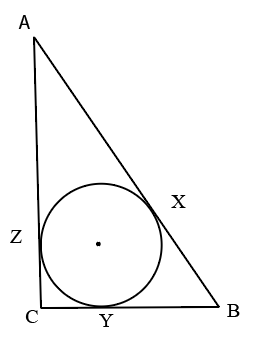Question 20

# A circle is inscribed in a right angled isosceles triangle. O is the centre of the circle which touches the triangle ABC at X, Y, Z. If $$AB=7\sqrt{2}$$ cm, then the ratio of AZ : BX : CY -Solution

If ACB is a right angled isosceles triangle and AB is hypotenuse with length 7*(root 2) length, then sides AC and BC = 7 cm
Now, the circle inscribed is an incircle.
Radius of in-circle = {7+7-[7*(root 2)]}/2 = $$7-\frac{7\sqrt{\ 2}}{2}$$

Now, if center of circle is marked as O, then quadrilateral OZCY is a square.
Hence, CY = CZ = $$7-\frac{7\sqrt{\ 2}}{2}$$

Hence, AZ = 7 - $$7-\frac{7\sqrt{\ 2}}{2}$$ $$=\frac{7\sqrt{\ 2}}{2}$$
Also, BX = BY and BY = BC - CY $$=\frac{7\sqrt{\ 2}}{2}$$
Hence, BX $$=\frac{7\sqrt{\ 2}}{2}$$

Hence, the given ratio becomes 1:1:[(root 2) - 1]